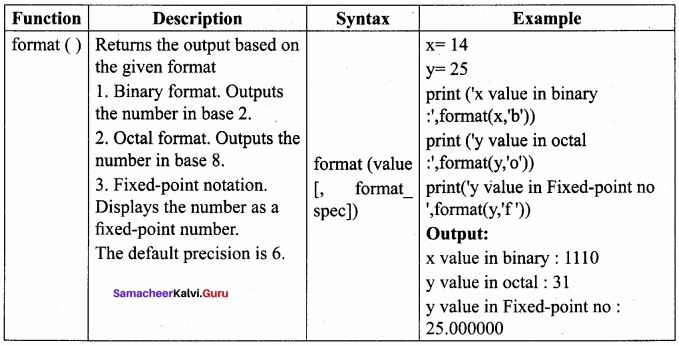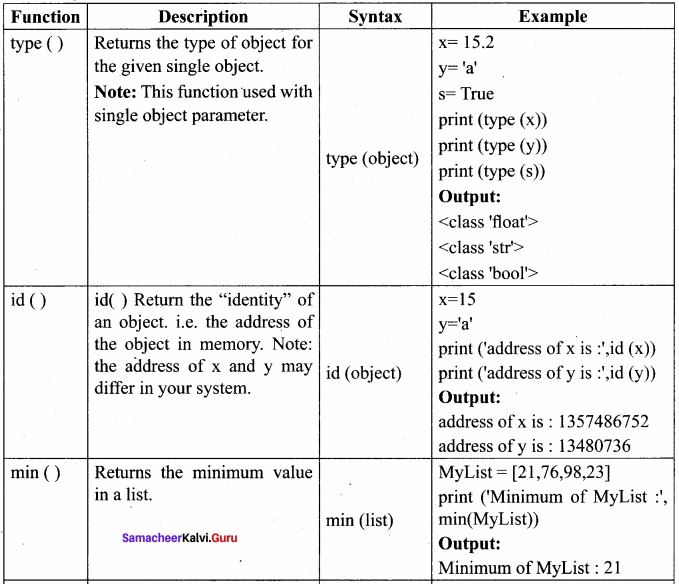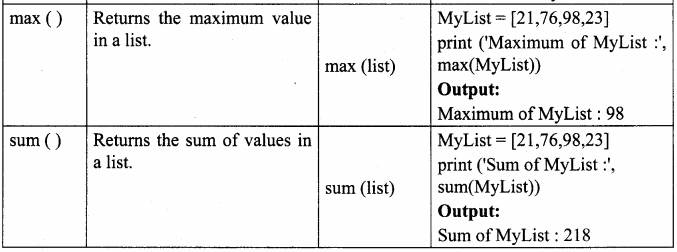# Samacheer Kalvi 12th Computer Science Solutions Chapter 7 Python Functions

Students can Download Computer Science Chapter 7 Python Functions Questions and Answers, Notes Pdf, Samacheer Kalvi 12th Computer Science Book Solutions Guide Pdf helps you to revise the complete Tamilnadu State Board New Syllabus and score more marks in your examinations.

## Tamilnadu Samacheer Kalvi 12th Computer Science Solutions Chapter 7 Python Functions

### Samacheer Kalvi 12th Computer Science Python Functions Text Book Back Questions and Answers

PART – I

12th Computer Science Chapter 7 Book Back Answers Question 1.
A named blocks of code that are designed to do one specific job is called as …………………………
(a) Loop
(b) Branching
(c) Function
(d) Block
(c) Function

Samacheer Kalvi Guru 12th Computer Science Question 2.
A Function which calls itself is called as …………………………
(a) Built – in
(b) Recursion
(c) Lambda
(d) Return
(b) Recursion

12th Computer Science Samacheer Question 3.
Which function is called anonymous un – named function?
(a) Lambda
(b) Recursion
(c) Function
(d) Define
(a) Lambda

Samacheer Kalvi Guru 7th Computer Science Question 4.
Which of the following keyword is used to begin the function block?
(a) Define
(b) For
(c) Finally
(d) Def
(d) Def

Computer Chapter 7 Question 5.
Which of the following keyword is used to exit a function block?
(a) Define
(b) Return
(c) Finally
(d) Def
(b) Return

7th Computer Guide Samacheer Kalvi Question 6.
While defining a function which of the following symbol is used.
(a) ; (semicolon)
(b) . (dot)
(c) : (colon)
(d) \$ (dollar)
(c) : (colon)

12th Computer Science Chapter 9 Book Back Answers Question 7.
In which arguments the correct positional order is passed to a function?
(a) Required
(b) Keyword
(c) Default
(d) Variable – length
(a) Required

Samacheer Kalvi Computer Science Book Question 8.
Read the following statement and choose the correct statement(s).
(I) In Python, you don’t have to mention the specific data types while defining function.
(II) Python keywords can be used as function name.

(a) (I) is correct and (II) is wrong
(b) Both are correct
(c) (I) is wrong and (II) is correct
(d) Both are wrong
(a) (I) is correct and (II) is wrong

Chapter 7 Science Question 9.
Pick the correct one to execute the given statement successfully.
if_: print (x,” is a leap year”)
(a) x % 2 = 0
(b) x % 4 = = 0
(c) x / 4 = 0
(d) x % 4 = 0
(b) x % 4 = = 0

Computer Science Samacheer Kalvi Question 10.
Which of the following keyword is used to define the function testpython():?
(a) Define
(b) Pass
(c) Def
(d) While
(c) Def

PART – II

Science Chapter 7 Question 1.
What is a function?
Functions are nothing but a group of related statements that perform a specific task.

Question 2.
Write the different types of functions?

1. User – defined Functions
2. Built-in Functions
3. Lambda Functions
4. Recursion Functions

Question 3.
What are the main advantages of function?

• It avoids repetition and makes a high degree of code reusing.
• It provides better modularity for your application.

Question 4.
What is meant by scope of variable? Mention its types?
Scope of variable refers to the part of the program, where it is accessible, i.e., area where you can refer (use) it. We can say that scope holds the current set of variables and their values. The two types of scopes are: local scope and global scope.

Question 5.
Define global scope?

• A variable, with global scope, can be used anywhere in the program.
• It can be created by defining a variable outside the scope of any function or block.

Question 6.
What is base condition in recursive function?
The condition that is applied in any recursive function is known as base condition. A base condition is must in every recursive function otherwise it will continue to execute like an infinite loop.

Question 7.
How to set the limit for recursive function? Give an example?
To set the limit for recursive function using sys.setrecursionlimit (limit _value).
Example:
import sys
sys. setrecursionlimit (3000)
def fact(n):
if n == 0:
return 1
else:
return n *
fact(n-l)
print(fact (2000)

PART – III

Question 1.
Write the rules of local variable?
Rules of local variable:

1. A variable with local scope can be accessed only within the function/block that it is created in.
2. When a variable is created inside the function/block, the variable becomes local to it.
3. A local variable only exists while the function is executing.
4. The formate arguments are also local to function.

Question 2.
Write the basic rules for global keyword in python?
The basic rules for global keyword in Python are:

1. When we define a variable outside a function, it’s global by default. You don’t have to use the global keyword.
2. We use global keyword to read and write a global variable inside a function.
3. Use of global keyword outside a function has no effect

Question 3.
What happens when we modify the global variable inside the function?
It shows an error, unbound local error.

Question 4.
Differentiate ceil ( ) and floor ( ) function?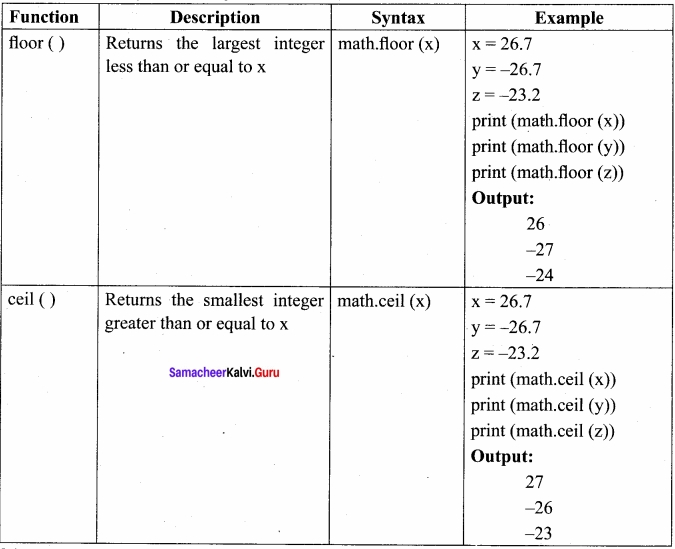Question 5.
Write a Python code to check whether a given year is leap year or not?
Leap year or not:
Program code:
y = int(input (“Enter year”))
if y%4==0:
print (“The given year is a leap year”)
else:
print (“The given year is not a leap year”)

Question 6.
What is a composition in functions?
The value returned by a function may be used as an argument for another function in a nested manner. This is called composition. For example, if we wish to take a numeric value or an expression as a input from the user, we take the input string from the user using the function input ( ) and apply eval ( ) function to evaluate its value.

Question 7.
How recursive function works?

• Recursive function is called .by some external code.
• If the base condition is met then the program gives meaningful output and exits.
• Otherwise, function does some required processing and then calls itself to continue recursion.

Question 8.
What are the points to be noted while defining a function?

1. Function blocks begin with the keyword “def ” followed by function name and parenthesis ( ).
2. Any input parameters or arguments should be placed within these parentheses when you define a function.
3. The code block always comes after a colon (:) and is indented.
4. The statement “return [expression]” exits a function, optionally passing back an expression to the caller. A “return” with no arguments is the same as return None

PART – IV

Question 1.
Explain the different types of function with an example?
Types of Functions:
Basically, we can divide functions into the following types:

1. User – defined Functions
2. Built – in Functions
3. Lambda Functions
4. Recursion Functions

Functions:
User – defined functions
Built – in functions
Lambda functions
Recursion functions

Description:
Functions defined by the users themselves.
Functions that are inbuilt with in Python.
Functions that are anonymous un-named function.
Functions that calls itself is known as recursive.

(I) Syntax for User defined function
def <function_name ( [parameter1, parameter!…] ) > :
<Block of Statements> return <expression /None>
Example:
def hello ( ):
print (“hello – Python”)
return

Advantages of User – defined Functions:

1. Functions help us to divide a program into modules. This makes the code easier to manage.
2. It implements code reuse. Every time you need to execute a sequence of statements, all you need to do is to call the function.
3. Functions, allows us to change functionality easily, and different programmers can work on different functions.

(II) Anonymous Functions:
In Python, anonymous function is a function that is defined without a name. While normal functions are defined using the def keyword, in Python anonymous functions are defined using the lambda keyword. Hence, anonymous functions are also called as lambda functions.

The use of lambda or anonymous function:

1. Lambda function is mostly used for creating small and one-time anonymous function.
2. Lambda functions are mainly used in combination with the functions like filter ( ), map ( ) and reduce ( ).

Syntax of Anonymous Functions
The syntax for anonymous functions is as follows:
lambda [argument(s)] expression
Example:
sum = lambda argl, arg2: argl + arg2
print (‘The Sum is sum (30, 40))
print (‘The Sum is :’, sum (-30, 40))
Output:
The Sum is: 70
The Sum is: 10
The above lambda function that adds argument argl with argument arg2 and stores the result in the variable sum. The result is displayed using the print ( ).

(III) Functions using libraries:
Built – in and Mathematical functions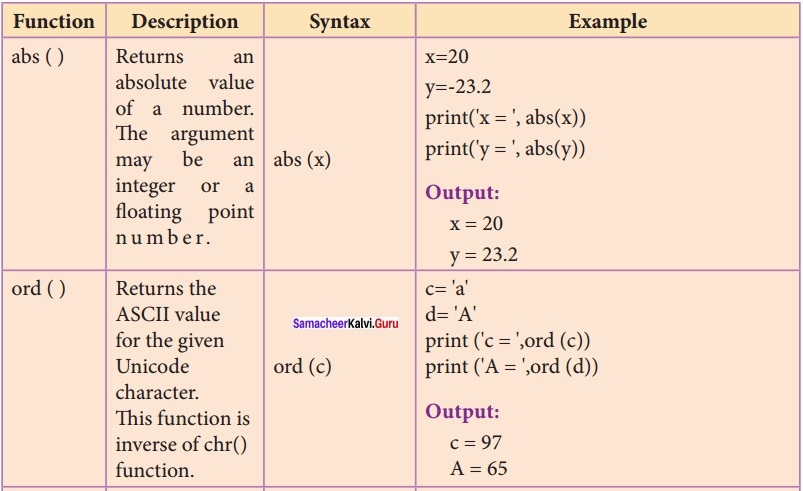(IV) Recursive functions:
When a function calls itself is known as recursion. Recursion works like loop but sometimes 1 ’ it makes more sense to use recursion than loop. You can convert any loop to recursion.
Example:
def fact(n):
if n = = 0:
return 1
else:
return n * fact (n – 1)
print (fact (0))
print (fact (5))
Output:
1
120

Question 2.
Explain the scope of variables with an example?
Scope of variable:

• Scope of variable refers to the part of the program, where it is accessible,
• The scope holds the current set of variables and their values.
• The two types of scopes are – local Scope and global scope

local Scope:

• A variable declared inside the function’s body or in the local scope is known as local variable.

Rules of local variable:

• A variable with local scope can be accessed only within the function/ block that it is created in.
• When a variable is created inside the function/block, the variable becomes local to it.
• A local variable only exists .while the function is executing.
• The format arguments are also local to function.

Example:
Create a Local Variable
def loc:
y=0 # local scope
print(y)
loc ()
Output:
0

Example:
Accessing local variable outside the scope
def loc():
y = “local”
loc()
print(y)

• When we run the above code, the output shows the following error:
• The above error occurs because we are trying to access a local variable ‘y’ in a global scope.

Name Error: name ‘y’ is not defined Global scope:
A variable, with global scope can be used anywhere in the program. It can be created by defining a variable outside the scope of any function/block.

Rules of global Keyword:

• When we define a variable outside a function, it’s global by default. You don’t have to use global keyword.
• We use global keyword to read and write a global variable inside a function.
• Use of global keyword outside a function has no effect.

Use of global keyword:
Example: Changing Global Variable From Inside a Function using global keyword
x = 0 # global variable
global x
x = x + 5 # increment by 2
function x value is :”, x)
print (“In main x value is :”, x)

Output:
Inside add() function x value is : 5
In main x value is : 5

• In the above program, x is defined as a global variable.
• Inside the add() function, the global keyword is used for x and we increment the variable x by 5.
• The change on the global variable x outside the function i.e the value of x is 5.

Question 3.
Explain the following built-in functions?
(a) id ( )
(b) chr ( )
(c) round ( )
(d) type ( )
(e) pow ( )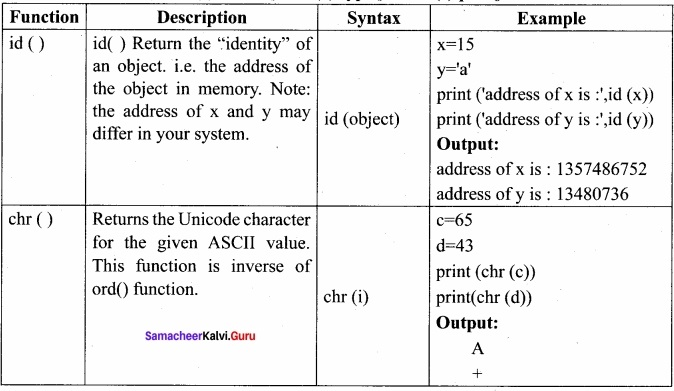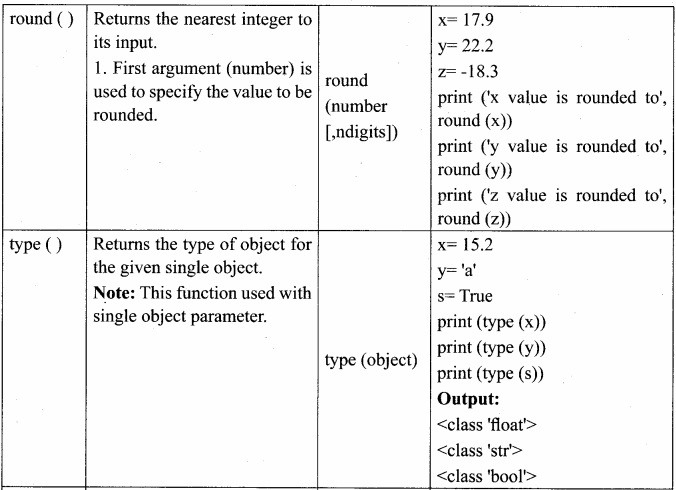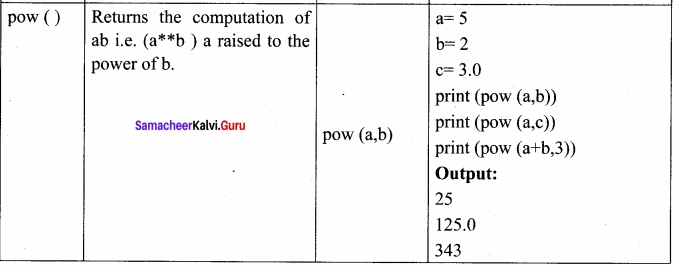Question 4.
Write a Python code to find the L.C.M. of two numbers?
Method I using functions:
def lcm (x, y):
if x > y:
greater = x
else:
greater = y while (true):
if ((greater % x = = 0) and (greater % y = = 0)):
lcm = greater break
greater + = 1
return lcm
num 1 = int (input(“Enter first number : “))
num 2 = int (input(“Enter second number : “))
print (“The L.C.M of”, numl, “and”, num, “is”, lcm(num1, num2))

Method II
(without using functions)
a = int (input (“Enter the first number :”))
b = int (input (“Enter the second number :”))
if a > b:
mini = a
else:
min 1 = b
while(1):
if (min 1 % a = = 0 and mini 1 % b = = 0):
print (“LCM is:”, mini)
break
mini = min 1 + 1
Output:
Enter the first number: 15
Enter the second number: 20
LCM is: 60

Question 5.
Explain recursive function with an example?
Recursive function:

• A recursive function calls itself. Imagine a process would iterate indefinitely if not stopped by some condition Such a process is known as infinite iteration.
• The condition that is applied in any recursive function is known as a base condition.
• A base condition is must in every recursive function otherwise it will continue to execute like an infinite loop.

Overview of how recursive function works:

• A recursive function is called by some external code.
• If the base condition is met then the. program gives meaningful output and exits.
• Otherwise, function does some required processing and then calls itself to continue recursion.
Here is an example of recursive function used to calculate factorial.

Example:
def fact(n):
if n==0:
return 1
else: ,
return n * fact (n-1)
print (fact (0))
print (fact (5))
Output:
1
120

Practice Programs

Question 1.
Try the following code in the above program?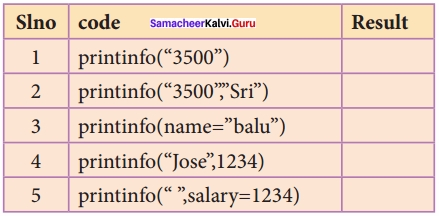Output:
1. Error

2. Name: Sri
Salary: 3500
Salary: 3500

3. Name: Balu
Salary: 3500

4. Name: Jose
Salary: 1234

5. Name:
Salary: 1234

Question 2.
Evaluate the following functions and write the output?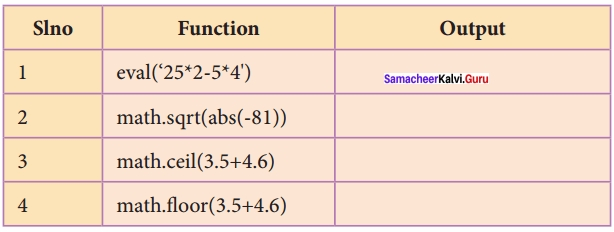Output:

1. 30
2. 9
3. 8
4. 9

Question 3.
Evaluate the following functions and write the output?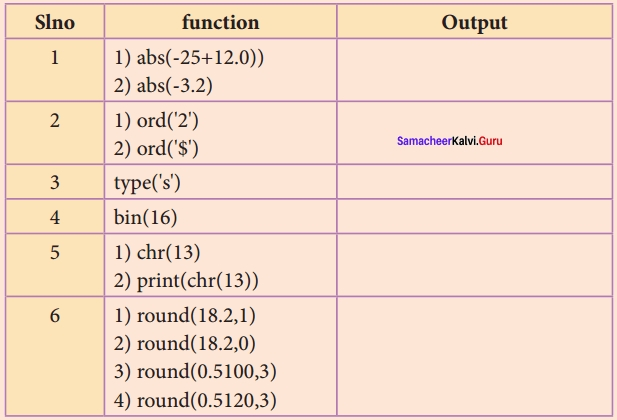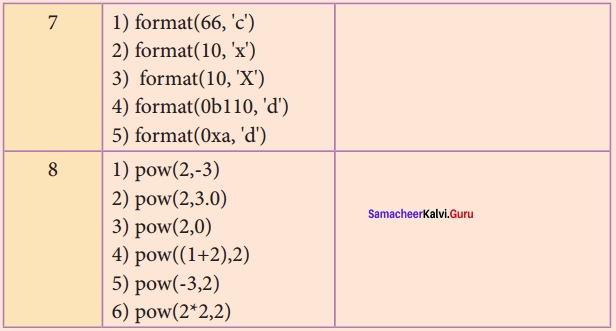Output:
(i) 1. 13
2. 3.2

(ii) 1. 50
2. 36

(iii) <class ‘str’>

(iv) 0b10000

(v). 1. CR (carriage return)
2. It moves the cursor to the beginning of the same line

(vi) 1. 8.2
2. 18.0
3. 0.510
4. 0.512

(vii) 1. B
2. a
3. A
4. 6
5. 10

(viii) 1. 0.125
2. 8.0
3. 1

### Samacheer Kalvi 12th Computer Science Python Functions Additional Questions and Answers

PART – 1

Question 1.
Which of the following avoids repetition and makes high degree of code reusing?
a) Loop
b) Functions
c) Branching
d) Dictionaries
b) Functions

Question 2.
A …………………………. is one or more lines of code, grouped together.
(a) Code –
(b) Block
(c) Function
(d) Arguments
(b) Block

Question 3.
A block of code begins when a line is indented by ……………………. spaces usually.
(a) 2
(b) 3
(c) 4
(d) 5
(c) 4

Question 4.
A block within a block is called …………………………… block.
Nested

Question 5.
How many types of arguments are used to call a function?
a) 3
b) 5
c) 4
d) 2
c) 4

Question 6.
How many types of function arguments are there?
(a) 2
(b) 3
(c) 4
(d) 5
(c) 4

Question 7.
The arguments can be given in improper order in ………………………. arguments.
(a) Required
(b) Keyword
(c) Default
(d) Variable – length
(b) Keyword

Question 8.
Which of the following keyword is used to define an anonymous function?
a) Def
b) Alpha
c) Range
d) Lambda
d) Lambda

Question 9.
How many methods of arguments passing are there in the variable-length method.
(a) 2
(b) 3
(c) 4
(d) 5
(a) 2

Question 10.
Non – keyword variable arguments are called ……………………………….
Tuples

Question 11.
In python’s ……………………….. function supports variable-length arguments.
(a) Input
(b) Write
(c) Output
(d) Print
(d) Print

Question 12.
Functions that are anonymous un_named are called ………………….
a) User-defined
b) Lambda
c) Built-in
d) Recursive
b) Lambda

Question 13.
Lambda function can only access ……………………….. variables.
(a) Local
(b) Function
(c) Global
(d) Nested
(c) Global

Question 14.
How many types of scopes are there?
(a) 2
(b) 3
(c) 4
(d) 5
(a) 2

Question 15.
Any loop can be converted into …………………….
a) Composition
b) Recursion
c) Function
d) Branching
b) Recursion

Question 16.
Read the following statement and choose the wrong statements
(a) Without using the global keyword, we cannot modify the global variable
(b) Using global keyword we can modify the global variable
(c) Without global keyword, we can modify the global variable
(c) Without global keyword, we can modify the global variable

Question 17.
Find the correct statement.
(a) Local and global variables cannot be used in the same code
(b) Local and global variables can be used in the same code
(b) Local and global variables can be used in the same code

Question 18.
The ……………………… function is the inverse of chr ( ) function.
(a) Ord ( )
(b) Abs ( )
(c) Chr ( )
(d) Bin ( )
(a) Ord ( )

Question 19.
…………………………. function is the alternative for bin ( ) function.
(a) Ord ( )
(b) Format ( )
(c) Binary ( )
(d) Ord ( )
(b) Format ( )

Question 20.
bin ( ) returns the binary string prefixed with ………………………… for the given integer number
(a) b
(b) ob
(c) obin
(d) bin
(b) ob

Question 21.
Find the output.
d = 43
print(‘A =ord(d))
(a) 67
(b) 95
(c) 97
(d) 65
(d) 65

Question 22.
Find the output,
d = 43
print (chr(d))
(a) –
(b) +
(c) *
(d) /
(b) +

Question 23.
The default precision for fixed-point number is ………………………….
(a) 2
(b) 4
(c) 6
(d) 8
(c) 6

Question 24.
How many formats are there for the format ( ) functions?
(a) 12
(b) 5
(c) 3
(d) 1
(c) 3

Question 25.
………………………. function returns the smallest integer greater than or equal to x
(a) Sqrt
(b) Flow
(c) Floor
(d) Cell
(d) Cell

Question 26.
………………………. function is used to evaluate the input value.
(a) Input
(b) Valuate
(c) Eval
(d) Val
(c) Eval

Question 27.
Any Loop can be converted to recursive functions.
True / False
True

Question 28.
Find true statement
(a) Recursive function call itself
(b) Recursive function have to be called externally
(a) Recursive function call itself

PART – II

Question 1.
What is meant by block in python?
A block is one or more lines of code, grouped together so that they are treated as one big sequence of statements while execution.

Question 2.
Give the syntax for passing parameters in functions?
Parameters or arguments can be passed to functions
def function _ name (parameter (s) separated by comma):

Question 3.
What are arguments? What are the types?

• Arguments are used to call a function and there are primarily four types of functions that one can use:
• Required arguments, Keyword arguments, Default arguments, and Variable-length arguments.

Question 4.
Classify Function Arguments?
Function Arguments:

1. Required arguments
2. Keyword arguments
3. Default arguments
4. Variable-length arguments

Question 5.
Define default arguments?
Default Arguments
In Python, the default argument is an argument that takes a default value if no value is provided in the function call. The following example uses default arguments, that prints default salary when no argument is passed, def printinfo(sal=3500):

Question 6.
Write the advantages of user-defined functions.

• Functions help us to divide a program into modules.
• This makes the code easier to manage.
• It implements code reuse. Every time you need to execute a sequence of statements, all you need to do is to call the function.
• Functions, allow us to change functionality easily, and different programmers can work on different functions.

PART – III

Question 1.
Write the output for the program given below?
Program:
def printdata (name, age):
print (“Example – 3 Keyword arguments”)
print (“Name :”,name)
print (“Age age)
return
# Now you can call printdata ( ) function
printdata (age = 25, name = “Gshan”)
Output:
Example – 3 Keyword arguments
Name: Gshan
Age: 25

Question 2.
Give the syntax for defining variable-length arguments.?
Syntax – Variable – Length Arguments:
def function _name (*args):
function_body
return_statement

Question 8.
Write a note of the return statement.

• The return statement causes your function to exit and returns a value to its caller.
• The point of functions, in general, is to take inputs and return something.
• The return statement is used when a function is ready to return a value to its caller.
• So, only one return statement is executed at run time even though the function contains multiple return statements.
• Any number of ‘return’ statements are allowed in a function definition but only one of them is executed at run time.

Question 4.
Find the output?
Program:
x = 0 # global variable
global x
x = x + 5 # increment by 2
print (“Inside add ( ) function x value is:”, x)
print (“In main x value is x)
Output:
Inside add ( ) function x value is: 5.
In main x value is: 5

Question 5.
Write note on format function?